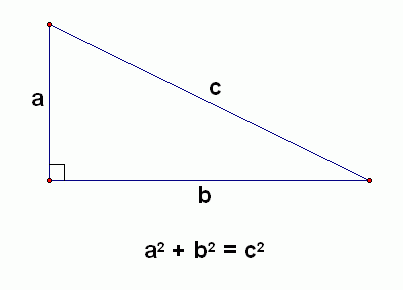# What is the distance between (9, 2, 0)  and (4, 3, 1) ?

Feb 29, 2016

$\sqrt{{\left(9 - 4\right)}^{2} + {\left(2 - 3\right)}^{2} + {\left(0 - 1\right)}^{2}} = \sqrt{{5}^{2} + {1}^{2} + {1}^{2}} = 3 \sqrt{3}$

#### Explanation:

The 2D Pythagorean Theorem states thatNow consider a 3D cuboid.Applying the 2D Pythagorean Theorem twice gives

${d}^{2} = {a}^{2} + {z}^{2} = \left({x}^{2} + {y}^{2}\right) + {z}^{2} = {x}^{2} + {y}^{2} + {z}^{2}$

Substituting the values $x = 5$, $y = 1$, $z = 1$ gives

${d}^{2} = {5}^{2} + {1}^{2} + {1}^{2} = 27$

$d = \sqrt{27} = 3 \sqrt{3}$

Feb 29, 2016

$3 \sqrt{3}$

#### Explanation:

The distance between any two points given the rectangular coordinates of the points is:
$\textcolor{w h i t e}{\text{XX}}$the square root of
$\textcolor{w h i t e}{\text{XXXX}}$the sum of
$\textcolor{w h i t e}{\text{XXXXXX}}$the squares of
$\textcolor{w h i t e}{\text{XXXXXXXX}}$the difference between each corresponding pair of coordinates.

In this case we have
{: ("point A",color(white)("XX"),"(",9,",",color(white)("X")2,",",color(white)("X")0,")"), ("point B",color(white)("XX"),"(",4,",",color(white)("X")3,",",color(white)("X")1,")"), ("difference",color(white)("XX"),"(",5,",",-1,",",-1,")"), ("square of diff",color(white)("XX"),"(",25,",",color(white)("X")1,",",color(white)("X")1,")") :}

distance $= \sqrt{25 + 1 + 1} = \sqrt{27} = 3 \sqrt{3}$